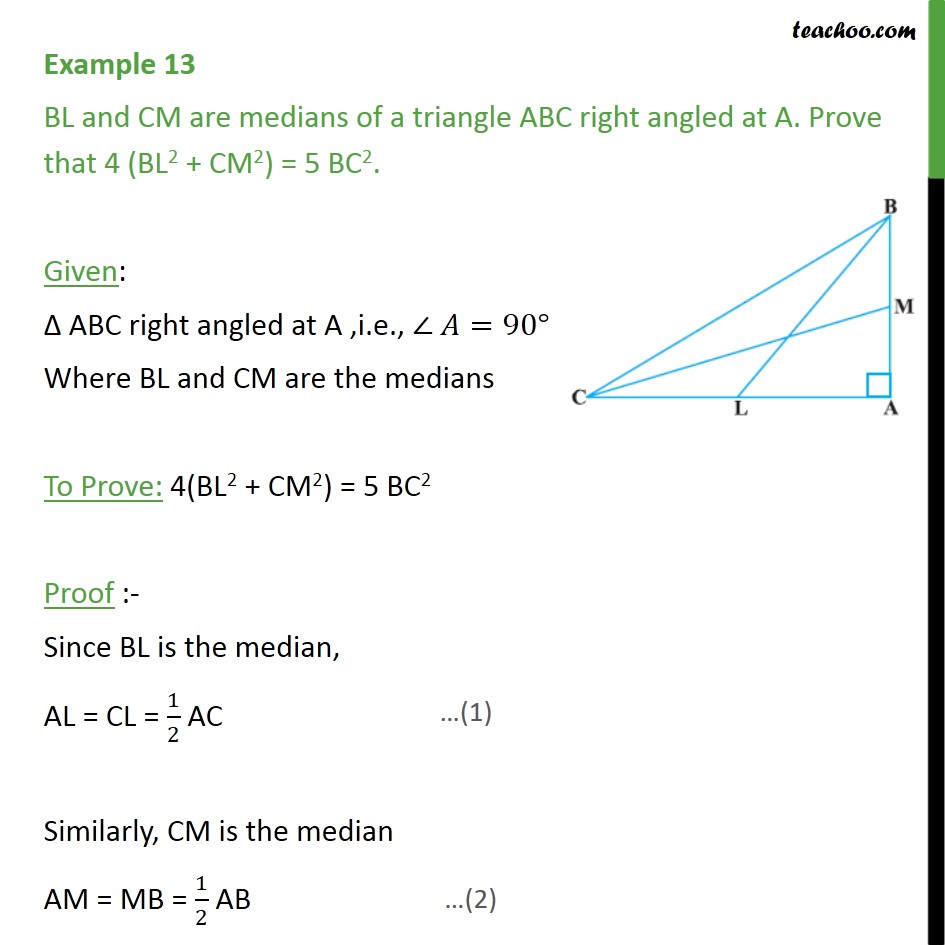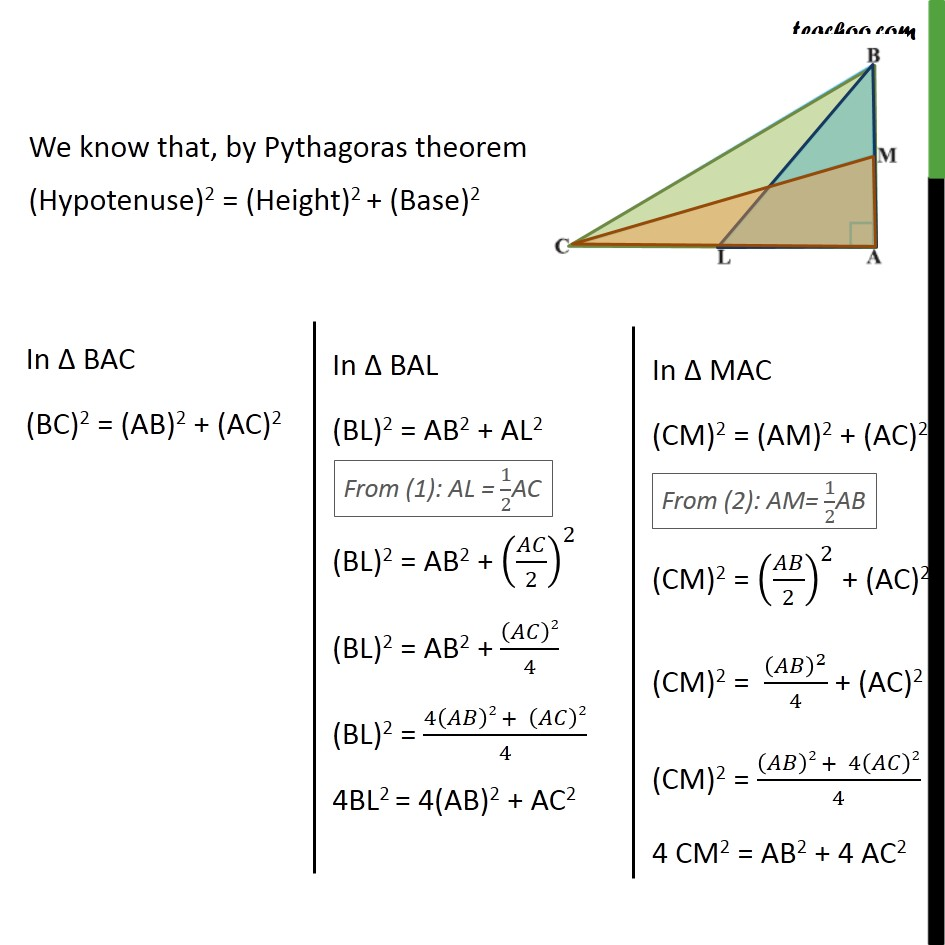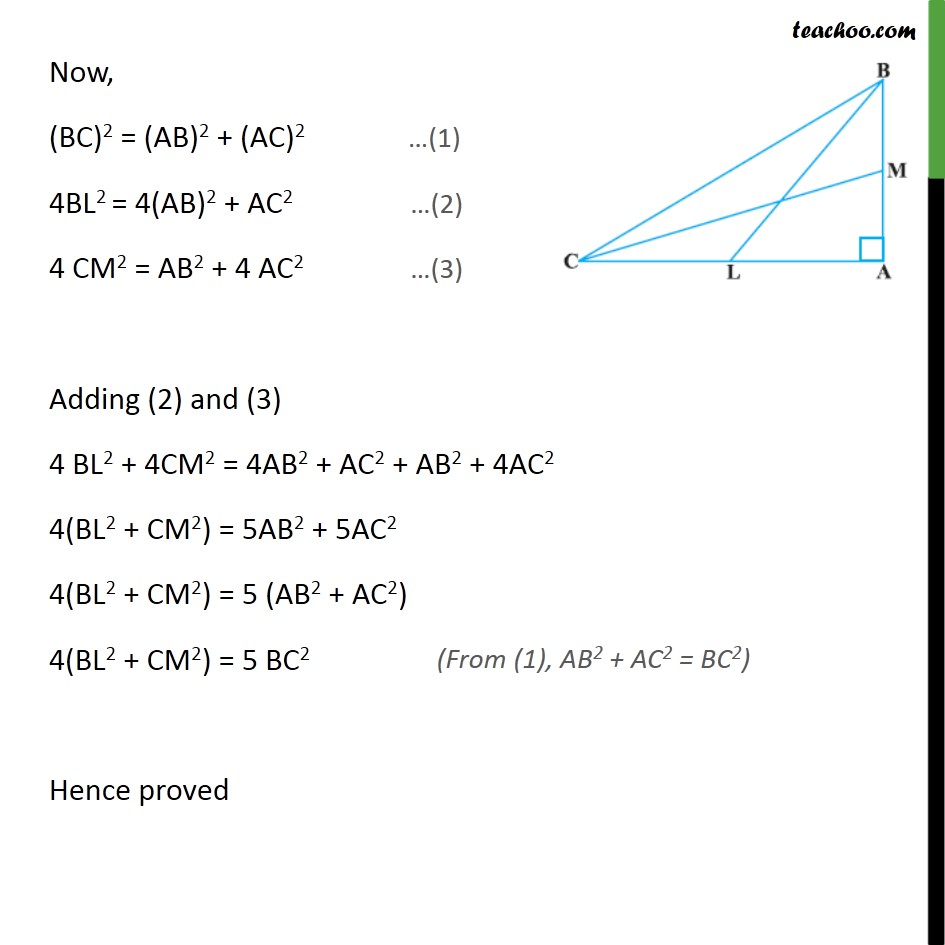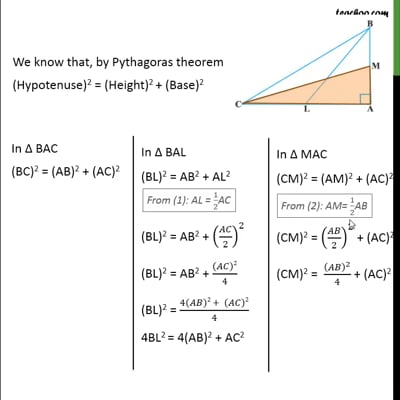Examples

Chapter 6 Class 10 Triangles (Term 1)
Serial order wiseThis video is only available for Teachoo black users

### Transcript

Example 13 BL and CM are medians of a triangle ABC right angled at A. Prove that 4 (BL2 + CM2) = 5 BC2. Given: Δ ABC right angled at A ,i.e., ∠ 𝐴=90° Where BL and CM are the medians To Prove: 4(BL2 + CM2) = 5 BC2 Proof :- Since BL is the median, AL = CL = 1/2 AC Similarly, CM is the median AM = MB = 1/2 AB We know that, by Pythagoras theorem (Hypotenuse)2 = (Height)2 + (Base)2 Now, (BC)2 = (AB)2 + (AC)2 …(1) 4BL2 = 4(AB)2 + AC2 …(2) 4 CM2 = AB2 + 4 AC2 …(3) Adding (2) and (3) 4 BL2 + 4CM2 = 4AB2 + AC2 + AB2 + 4AC2 4(BL2 + CM2) = 5AB2 + 5AC2 4(BL2 + CM2) = 5 (AB2 + AC2) 4(BL2 + CM2) = 5 BC2 Hence proved